# PSEB 12th Class Chemistry Solutions Chapter 1 The Solid State

Punjab State Board PSEB 12th Class Chemistry Book Solutions Chapter 1 The Solid State Textbook Exercise Questions and Answers.

## PSEB Solutions for Class 12 Chemistry Chapter 1 The Solid State

### PSEB 12th Class Chemistry Guide The Solid State InText Questions and Answers

Question 1.
Define the term ‘amorphous’. Give a few examples of amorphous solids.
Amorphous solids are the solids whose constituent particles are of irregular shapes and have short range order. In such an arrangement, a regular and periodically repeating pattern is observed over short distances only. Examples are glass, rubber, and plastic.

Question 2.
What makes a glass different from a solid such as quartz? Under what conditions could quartz be converted into glass?
Glass is an amorphous solid in which the constituent particles (SiO4 tetrahedral) have only a short range order, but in quartz, the constituent particles have both long range and short range orders. Quartz can be converted into glass by melting it and cooling it rapidly.

Question 3.
Classify each of the following solids as ionic, metallic, molecular, network (covalent) or amorphous.
(i) Tetra phosphorus decoxide (P4O10)
(ii) Ammonium phosphate (NH4)3PO4
(iii) SiC
(iv) I2
(v) P4
(vi) Plastic
(vii) Graphite
(viii) Brass
(ix) Rb
(x) LiBr (xi) Si.
Ionic Solid – (ii) Ammonium phosphate (NH4)3PO4, (x) LiBr
Metallic Solid – (viii) Brass, (ix) Rb
Molecular Solid – (i) Tetra phosphorus decoxide (P4O10), (iv) I2, (v) P4.
Covalent (network) Solid – (iii) SiC, (vii) Graphite, (xi) Si
Amorphous Solid – (vi) PlasticQuestion 4.
(i) What is meant by the term ‘coordination number’?
(ii) What is the coordination number of atoms:
(a) in a cubic close-packed structure?
(b) in a body-centred cubic structure?
(i) The number of nearest neighbours of any constituent particle present in the crystal lattice is called its coordination number.
(ii) The coordination number of atoms
(a) in a cubic close-packed structure is 12, and
(b) in a body-centred cubic structure is 8

Question 5.
How can you determine the atomic mass of an unknown metal if you know its density and the dimension of its unit cell? Explain.
Atomic mass of element, M = $$\frac{d a^{3} N_{A}}{z}$$
where, d = density
z = Number of atoms present in one unit cell.

Question 6.
‘Stability of a crystal is reflected in the magnitude of its melting point’. Comment. Collect melting points of solid water, ethyl alcohol, diethyl ether and methane from a data book. What can you say about the intermolecular forces between these molecules?
(i) Higher the melting point, greater is the intermolecular force of attraction and greater is the stability. A substance with higher melting point is more stable than a substance with lower melting point.

(ii) The melting points of the given substances are: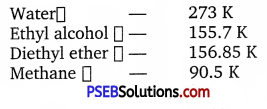The intermolecular forces in molecules of water and ethyl alcohol are mainly hydrogen bonding. The magnitude is more in water than in ethyl alcohol which is evident from the value of their melting points. The intermolecular forces in the molecules of diethyl ether are dipolar forces while in methane only weak vander waals’ force of attraction exist. The value of melting points are the evidences for the same.Question 7.
How will you distinguish between the following pairs of terms:
(i) Hexagonal close-packing and cubic close-packing?
(ii) Crystal lattice and unit cell?
(iii) Tetrahedral void and octahedral void?
(i) Hexagonal close packing (hep): The first layer is formed utilizing maximum space, thus wasting minimum space. In every second row the particles occupy the depressions (also called voids) between the particles of the first row. In the third row, the particles are vertically aligned with those in the first row giving AB AB AB … arrangement. This structure has hexagonal symmetry and is known as hexagonal close packing (hep). This packing is more efficient and leaves small space which is unoccupied by spheres. In hep arrangement, the coordination number is 12 and only 26% space is free. A single unit cell has 4 atoms.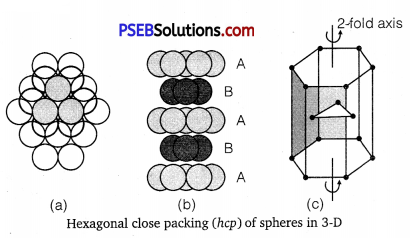Cubic close packing (ccp) Again, if we start with hexagonal layer of spheres and second layer of spheres is arranged by placing the spheres over the voids of the first layer, half of these holes can be filled by these spheres. Presume that spheres in the third layer are arranged to cover octahedral holes. This arrangement leaves third layer not resembling with either first or second layer, but fourth layer is similar to first, fifth
layer to second, sixth to third and so on giving pattern ABCABCABC …. This arrangement has cubic symmetry and is known as cubic closed packed (ccp) arrangement. This is also called face-centred cubic (fee) arrangement.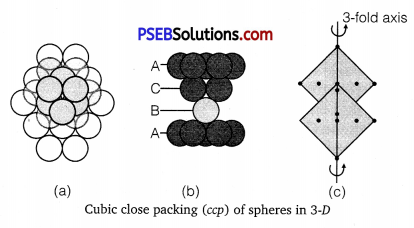The free space available in this packing is 26% and coordination number is 12.

(ii) The regular three dimensional arrangement of identical points in the space which represent how the constituent particles (atoms, ions, molecules) are arranged in a crystal is called a crystal lattice.
A unit cell is the smallest portion of a crystal lattice, which when repeated over and again in different directions produces the complete crystal lattice.

(iii) A void surrounded by four spheres occupying the corners of tetrahedron is called a tetrahedral void. It is much smaller than the size of spheres in the close packing. A void surrounded by six spheres along the corners of an octahedral is called octahedral void. The size of the octahedral void is smaller than that of the spheres in the close packing but larger than the tetrahedral void.

Question 8.
How many lattice points are there in one unit cell of each of the following lattice?
(i) Face-centred cubic
(ii) Face-centred tetragonal
(iii) Body-centred
Solution:
(i) Number of corner atoms per unit cell
= 8 corners × $$\frac{1}{8}$$ atom per unit cell 8
= 8 × $$\frac{1}{8}$$ = 1 atom 8
Number of face centred atoms per unit cell
= 6 face centred atoms × $$\frac{1}{2}$$ atom per unit cell
= 6 × $$\frac{1}{2}$$ = 3 atoms
∴ Total number of atoms or lattice points =1 + 3 = 4

(ii) As in (i) ;
No. of lattice points = 4

(iii) In bcc unit cell, number of comer atoms per unit cell
= 8 corners × $$\frac{1}{8}$$ per corner atom 8
= 8 × $$\frac{1}{8}$$ = 1 atom 8
Number of atoms at body centre = 1 × 1 = 1 atom
∴ Total number of atoms or lattice points = 1 + 1 = 2

Question 9.
Explain
(i) The basis of similarities and differences between metallic and ionic crystals.
(ii) Ionic solids are hard and brittle.
(i) Similarities
(a) Both ionic and metallic crystals have electrostatic forces of attraction. In ionic crystals, these are between the oppositely charged ions. In metals, these are among the valence electrons and the kernels.
(b) In both cases, the bond is non-directional.

Differences
(a) In ionic crystals, the ions are not free to move. Hence, they cannot conduct electricity in the solid state. They can do so only in the molten state or in aqueous solution. In metals, the valence electrons are not bound but are free to move. Hence, they can conduct electricity in the solid state.

(b) Ionic bond is strong due to electrostatic forces of attraction. Metallic bond may be weak or strong depending upon the number of valence electrons and the size of the kernels.

(ii) Ionic crystals are hard because there are strong electrostatic forces of attraction among the oppositely charged ions. They are brittle because ionic bond is non-directional.Question 10.
Calculate the efficiency of packing in case of a metal crystal for
(i) simple cubic
(ii) body-centred cubic
(iii) face-centred cubic (with the assumptions that atoms are touching each other).
Solution: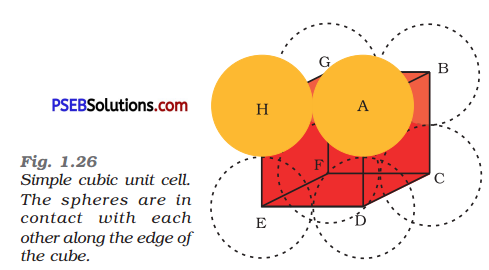(i) Simple Cubic: In a simple cubic lattice, the particles are located only at the corners of the cube and touch each other along the edge.
Let the edge length of the cube be ‘a’ and the radius of each particle be r.
So, we can write: a = 2r
Now, volume of the cubic unit cell = a3
= (2r)3
= 8r3
We know that the number of particles per unit cell is 1.
Therefore, volume of the occupied unit cell
= $$\frac{4}{3}$$ πr3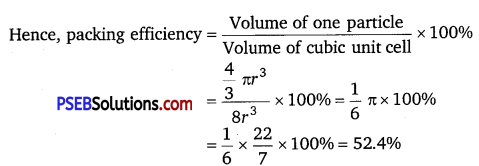(ii) Body-centred cubic: It can be observed from the figure given below that the atom at the centre is in contact with the other two atoms diagonally arranged.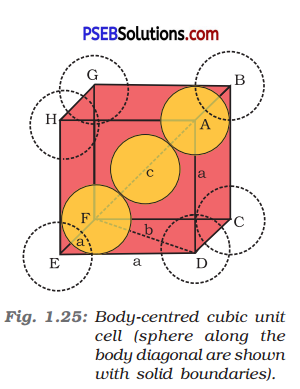From ΔFED, we have
b2 = a2 + a2
⇒ b2 = 2a2
⇒ b = √2a
Again, from ΔAFD, we have
c2 = a2 + b2
⇒ c2 = a2 + 2a2 (since b2 = 2a2)
⇒ c2 = 3a2
⇒ c = √3a
Let the radius of the atom be r.
Length of the body diagonal, c = 4r
⇒ √3a = 4 r
⇒ a = $$\frac{4 r}{\sqrt{3}}$$
r = $$\frac{\sqrt{3} a}{4}$$
or Volume of the unit cell a3 = ($$\frac{4 r}{\sqrt{3}}$$)3
A body-centred cubic lattice contains 2 atoms.
So, volume of the occupied cubic lattice = 2 x $$\frac{4}{3}$$ r3
= $$\frac{8}{3}$$πr3
∴ Packing efficiency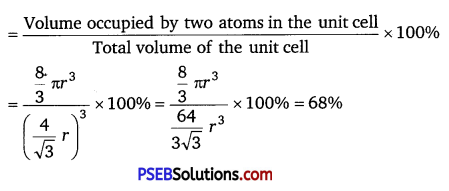(iii) Face-centred cubic: Let the edge length of the unit cell be ‘a’ and the length of the face diagonal AC be b.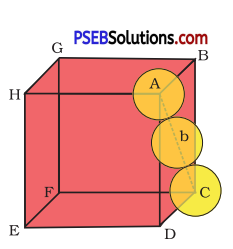From AABC, we have
AC2 = BC2 + AB2
⇒ b2 = a2 + a2
⇒ b2 = 2a2
⇒ b = √2a
⇒ 4r = √2a ⇒ = $$\frac{4 r}{\sqrt{2}}$$ (∵ b = 4r)
Volume of the unit cell, a3 = ($$\frac{4 r}{\sqrt{2}}$$)3
A face-centred cubic lattic contains 4 atoms
So, volume of the occupied cubic lattic = 4 × $$\frac{4}{3}$$ πr3 = $$\frac{16}{3}$$ πr3
∴ Packing efficiency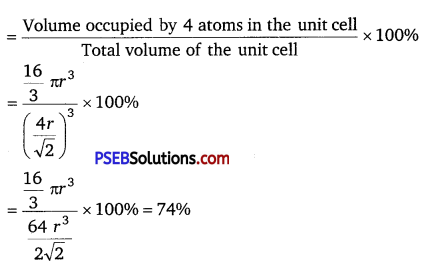Question 11.
Silver crystallises in fee lattice. If edge length of the cell is 4.07 × 10-8 cm and density is 10.5 g cm-3, calculate the atomic mass of silver.
Solution:
Given, a = 4.07 × 10-8 cm, d = 10.5 g cm-3
Number of atoms in fee lattice (z) = 8 × $$\frac{1}{8}$$ + 6 × $$\frac{1}{2}$$ = 1 + 3 = 4
We also know that, NA = 6.022 × 1023 mol-1 (Avogadro’s constant)
Using the formula
d = $$\frac{z M}{a^{3} N_{A}}$$
M = $$\frac{d a^{3} N_{\cdot A}}{z}$$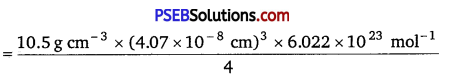= 107.13 g mol-1
Hence, atomic mass of silver = 107.13.Question 12.
A cubic solid is made of two elements P and Q. Atoms of Q are at the comers of the cube and P at the body-centre. What is the formula of the compound? What are the coordination numbers ofPandQ?
Solution:
It is given that the atoms of Q are present at the corners of the cube.
Therefore, number of atoms of Q in one unit cell = 8 × $$\frac{1}{8}$$ = 1
It is also given that the atoms of P are present at the body-centre.
Therefore, number of atoms of P in one unit cell = 1
This means that the ratio of the number of P atoms to the number of Q
atoms, P : Q = 1 : 1
Hence, the formula of the compound is PQ.
The coordination number of both P and Q is 8.

Question 13.
Niobium crystallises in body-centred cubic structure. If density is 8.55 g cm-3, calculate atomic radius of niobium using its atomic mass 93 u.
Solution:
Given, d. = 8.55 g cm-3, M = 93gmol-1
Number of atoms in bcc lattice (z) = 8 × $$\frac{1}{8}$$ + 1 × 1 = 1 + 1 = 2
We know that, NA = 6.022 × 10 23 mol-1 (Avogadro’s constant)
Using the formula
d = $$\frac{z M}{a^{3} N_{A}}$$
⇒ a3 = $$\frac{z M}{d N_{A}}$$ = $$\frac{2 \times 93 \mathrm{~g} \mathrm{~mol}^{-1}}{8.55 \mathrm{gcm}^{-3} \times 6.022 \times 10^{23} \mathrm{~mol}^{-1}}$$
= 3.612 × 10-23 cm3
so, a = 3.306 × 10-8cm
For body-centerd cubic unit cell:
r = $$\frac{\sqrt{3}}{4}$$ a = $$\frac{\sqrt{3}}{4}$$ × 3.306 10-8cm
= 1.432 × 10-8 cm = 14.32 × 10-6 cm = 14.32

Question 14.
If the radius of the octahedral void is r and radius of the atoms in close packing is R, derive relation between r and R.
Solution:
A sphere with centre 0, is fitted into the octahedral void as shown in the figure given below. It can be observed from the figure that ΔPOQ is right-angled
∠POQ =90°
Now, applying Pythagoras theorem, we have
PQ2 = PO2 + OQ2
⇒ (2R)2 = (R + r2) + (R + r)2
⇒ (2R)2 = 2(R + r)
⇒ 2R2 = (R + r)2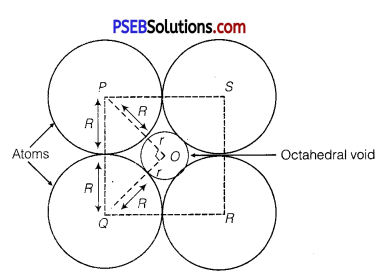⇒ √R = R + r
⇒ r = √2R – R
⇒ r = (√2 – 1)K
⇒ r = (1.414 – 1)R
⇒ r = 0.4141 RQuestion 15.
Copper crystallises into a fee lattice with edge length 3.61 × 10-8 cm. Show that the calculated density is in agreement with its measured value of 8.92 g cm-3.
Solution:
Given, edge length, a = 3.61 × 10-8 cm
Number of atoms of Cu in fee unit cell, z = 8 × $$\frac{1}{8}$$ + 6 × $$\frac{1}{2}$$ = 1 + 3 = 4
Atomic mass, M = 63.5 g mol-1
We know that, NA = 6.022 × 1023 mol-1 (Avogadro’s number)
Using the formula
d = $$\frac{z M}{a^{3} N_{A}}$$
= $$\frac{4 \times 63.5 \mathrm{~g} \mathrm{~mol}^{-1}}{\left(3.61 \times 10^{-8} \mathrm{~cm}\right)^{3} \times\left(6.022 \times 10^{23} \mathrm{~mol}^{-1}\right)}$$
= 8.97 g cm
The measured value of density is given as 8.92 g cm-3 . Hence, the calculated density 8.97 g cm-3 is in agreement with its measured value.

Question 16.
Analysis shows that nickel oxide has the formula Ni0.98O1.00.
What fractions of nickel exist as Ni2+ and Ni3+ions?
Solution:
98 Ni atoms are associated with 100 O atoms. Out of 98 Ni atoms,
suppose Ni present as Ni2+ = x
Then, Ni present as Ni3+ = 98 – x
Total charge on x Ni2+ and (98 – x) Ni3+ should be equal to charge on 100 O2- ions.
Therefore x × 2 + (98 – x) × 3 = 100 × 2
2x + 294 – 3x = 200
x = 94
∴ Fraction of Ni present as Ni2+ = $$\frac{94}{98}$$ × 100 = 96%
Fraction of Ni present as Ni3+ = $$\frac{4}{98}$$ × 100 = 4%

Question 17.
What is a semiconductor? Describe the two main types of semiconductors and contrast their conduction mechanism.
Semiconductors are substances having conductance in the intermediate range of 10-6 to 104 ohm-1 m-1. As there is rise in the temperature, conductivity also increase because electrons from the valence band jump to conduction band.
The two main types of semiconductors are:
n-type semiconductor: The semiconductor whose increased conductivity is a result of negatively-charged electrons is called an n-type semiconductor. When the crystal of a group 14 element such as Si or Ge is doped with a group 15 element such as P or As, an n-type semiconductor is formed.

Si and Ge have four valence electrons each. In their crystals, each atom forms four covalent bonds. On the other hand, P and As contain five valence electrons each. When Si or Ge is doped with P or As, the latter occupies some of the lattice sites in the crystal. Four out of five electrons are used in the formation of four covalent bonds with four neighbouring Si or Ge atoms. The remaining fifth electron becomes delocalised and increases the conductivity of the doped Si or Ge.

p-type semiconductor: The semiconductor whose increased in conductivity is a result of electron hole is called a p-type semiconductor. When a crystal of group 14 elements such as Si or Ge is doped with a group 13 element such as B, Al, or Ga (which contains only three valence electrons), a p-type of semiconductor is formed.

When a crystal of Si is doped with B, the three electrons of B are used in the formation of three covalent bonds and an electron hole is created. An electron from the neighbouring atom can come and fill this electron hole, but in doing so, it would leave an electron hole at its original position. The process appears as if the electron hole has moved in the direction opposite to that of the electron that filled it. Therefore, when an electric field is applied, electrons will move toward the positively-charged plate through electron holes. However, it will appear as if the electron holes are positively-charged and are moving toward the negatively- charged plate.Question 18.
Non-stoichiometric cuprous oxide, Cu2O can he prepared in laboratory. In this oxide, copper to oxygen ratio is slightly less than 2:1. Can you account for the fact that this substance is a p-type semiconductor?
In the cuprous oxide (Cu2O) prepared in the laboratory, copper to oxygen ratio is slightly less than 2:1. This means that the number of Cu+ ions is slightly less than twice the number of O2- ions. This is because some Cu+ ions have been replaced by Cu2+ ions. Every Cu2+ ion replaces two Cu+ ions, thereby creating holes. As a result, the substance conducts electricity with the help of these positive holes. Hence, the substance is a p-type semiconductor.

Question 19.
Ferric oxide crystallises in a hexagonal close-packed array of oxide ions with two out of every three octahedral holes occupied by ferric ions. Derive the formula of the ferric oxide.
Solution:
Let the number of oxide ions (O2-) in the closed packing be x.
So, number of octahedral voids = x
It is given that two out of every three octahedral holes are occupied by ferric ions.
So, number of ferric (Fe3+ ) ions = $$\frac{2}{3}$$x
Therefore, ratio of the number of Fe3+ ions to the number of O2- ions,
Fe3+: O2- = $$\frac{2}{3}$$x : x = $$\frac{2}{3}$$ : 1 = 2 : 3
Hence, the formula of the ferric oxide is Fe2O3.

Question 20.
Classify each of the following as being either a p-type or an n-type semiconductor:
(i) Ge doped with In
(ii) Si doped with B.
(i) Ge (a group 14 element) is doped with In (a group 13 element). Therefore, a hole will be created and the semiconductor generated will be a p-type semiconductor.
(ii) Si (a group 14 element) is doped with B (a group 13 element). Thus, a hole will be created and the semiconductor generated will be a p-type semiconductor.

Question 21.
Gold (atomic radius = 0.144 nm) crystallises in a face-centred unit cell. What is the length of a side of the cell?
Solution:
For a face-centred unit cell (fee)
Edge length, (a) = 2√2r
It is given that the atomic radius, r = 0.144 nm
So, a = 2√2 × 0.144 nm = 0.407 nm
Hence, length of a side of the cell = 0.407 nmQuestion 22.
In terms of band theory, what is the difference (i) between a conductor and an insulator
between a conductor and a semiconductor
(i) The energy gap between the valence band and conduction band in an insulator is very large while in a conductor, the energy gap is very small or there is overlapping between valence band and conduction band.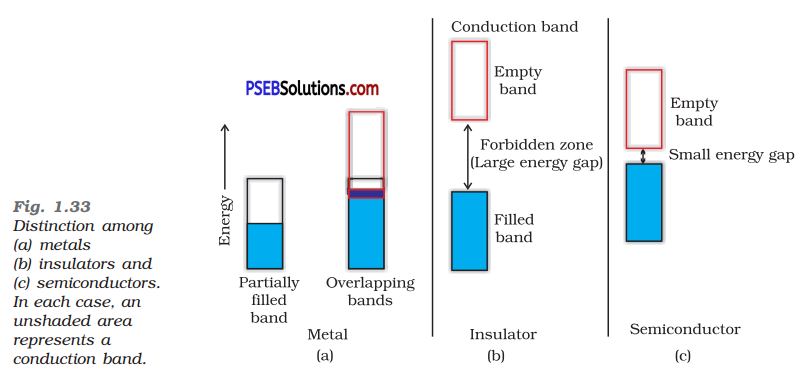(ii) In a conductor, there is a very small energy gap or there is overlapping between valence band and conduction band whereas in semiconductor there is always a small energy gap between them.

Question 23.
Explain the following terms with suitable examples:
(i) Schottky defect
(ii) Frenkel defect
(iii) Interstitials and
(iv) F-centres
(i) Schottky defect: This defect arises when equal number of cations and anions are missing from the lattice. It is a common defect in ionic compounds of high coordination number where both cations and anions are of the same size, e.g., KCl, NaCl, KBr, etc. Due to this defect, density of crystal decreases and it begins to conduct electricity to a smaller extent.(ii) Frenkel defect: This defect arises when some of the ions of the lattice occupy interstitial sites leaving lattice sites vacant. This defect is generally found in ionic crystals where anion is much larger in size than the cation, e.g., AgBr, ZnS, etc. Due to this defect density does not change, electrical conductivity increases to a small extent and there is no change in over all chemical composition of the crystal.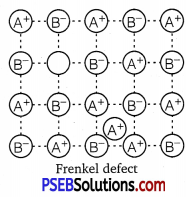(iii) Interstitial defect : When some constituent particles (atoms or molecules) occupy an interstitial site, the crystal is said to have interstitial defect. Due to this defect the density of the substance increases.
Vacancy and interstitial defects are generally shown by non-ionic solids because ionic solids must always maintain electrical neutrality.

(iv) F-centres: These are the anionic sites occupied by unpaired electrons. F-centres impart colour to crystals. They impart yellow colour to NaCl crystals, violet colour to KCl crystals and pink colour to LiCl crystals.
The colour results by the excitation of electrons when they absorb energy from the visible light falling on the crystal.

Question 24.
Aluminium crystallises in a cubic close-packed structure. Its metallic radius is 125 pm.
(i) What is the length of the side of the unit cell?
(ii) How many unit cells are there in 1.00 cm3 of aluminium?
Solution:
(i) For an fee unit cell, r = $$\frac{a}{2 \sqrt{2}}$$ (given, r = 125 pm)
a = 2√2 r = 2√2 × 125 pm
= 353.55 pm
≅354 pm

(ii) Volume of one unit cell = a3 = (354 pm)3
= 4.4 × 107 pm3
= 4.4 × 107 × 10-30cm3
= 4.4 × 10-23 cm3
Therefore, number of unit cells in 1.00 cm3 = $$\frac{1.00 \mathrm{~cm}^{3}}{4.4 \times 10^{-23} \mathrm{~cm}^{3}}$$
= 2.27 × 1022Question 25.
If NaCl is doped with 10-3 mol % of SrCl2, what is the concentration of cation vacancies?
Solution:
It is given that NaCl is doped with 10-3 mol% of SrCl2, this means that 100 mol of NaCl are doped with 10-3 mol of SrCl2.
Therefore, 1 mol of NaCl is doped with SrCl2 = $$\frac{10^{-3}}{100}$$ = 10-5 mol
Cation vacancies produced by one Sr2+ ion = 1
∴ Concentration of the cation vacancies produced by 10-5 mol of Sr2+ ions
= 10-5 × 6.022 × 1023
= 6.022 × 1018 mol-1
Hence, the concentration of cation vacancies created by SrCl2 is 6.022 × 1018 per mol of NaCl.

Question 26.
Explain the following with suitable examples:
(i) Ferromagnetism
(ii) Paramagnetism
(iii) Ferrimagnetism
(iv) Antiferromagnetism
(v) 12-16 and 13-15 group compounds.
(i) Ferromagnetism: They have strong attraction towards the magnetic field. These substances can be permanently magnetised.
In solid state, the metal ions of ferromagnetic substances are grouped together into small regions, called domains. Thus, each domain acts as a tiny magnet. When the substance is placed in a magnetic field all the domains get oriented in the direction of the magnetic field, and a
strong magnetic effect is produced. This ordering domains persist even when the magnetic field is removed and the ferromagnetic substance becomes a permanent magnet.
A few substances like iron, cobalt, nickel, CrO2 shows ferromagnetism at room temperature.(ii) Paramagnetism: These materials are weakly attracted by a magnetic field. They can be magnetised in a magnetic field in the same direction Paramagnetism is due to the presence of one or more unpaired electrons which are attracted by the magnetic field.
O2, Cu2+, Fe3+, Cr3+ are some examples of such substances. They loss their magnetism in the absence of magnetic field.

(iii) Ferrimagnetism: When the magnetic moments of the domains in the substance are aligned in parallel and antiparallel direction in unequal numbers they are weakly attracted by magnetic field as compared to ferromagnetic substances. Fe3O4 (magnetite) and ferrites like MgFe2O4 and ZnFe2O4 are examples of such substances. These substances also lose ferrimagnetism on heating and become paramagnetic.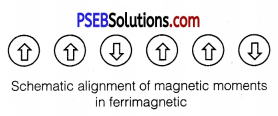(iv) Antiferromagnetism: In these substances their domains are aligned in such a way that net magnetic moment is zero. This type of magnetism is called antiferromagnetism. For example, MnO has antiferromagnetism.

(v) 12-16 and 13-15 group compounds: Combination of elements of groups 12 and 16 yield some solid compounds which are referred to as 12-16 compounds. For example, ZnS, CdS, CdSe, HgTe, etc. In these compounds, the bonds have ionic character.
When the solid state materials are produced by combination of elements of groups 13 and 15, the compounds thus obtained are called 13-15 compounds. For example, InSb, AIP, GaAs, etc.### Chemistry Guide for Class 12 PSEB The Solid State Textbook Questions and Answers

Question 1.
Why are solids rigid?
The intermolecular forces of attraction that are present in solids are very strong. The constituent particles of solids cannot move from their positions i.e., they have fixed positions. They can only oscillate about their mean positions due to strong attraction forces between the particles. This imparts rigidity.

Question 2.
Why do solids have a definite volume?
The constituent particles in solids are bound to their mean positions by strong forces of attraction. The interparticle distances remain unchanged at a given temperature. Therefore, solids have a definite volume.

Question 3.
Classify the following as amorphous or crystalline solids:
Polyurethane, naphthalene, benzoic acid, teflon, potassium nitrate, cellophane, polyvinyl chloride, fibre glass, copper.
Amorphous solids Polyurethane, teflon, cellophane, polyvinyl chloride, fibre glass.
Crystalline solids: Naphthalene, benzoic acid, potassium nitrate, copper

Question 4.
Why is glass considered a super cooled liquid?
Similar to liquids, glass has a tendency to flow, though very slowly. Therefore, glass is considered as a super cooled liquid. This is the reason that glass windows or doors of old buildings are invariably found to be slightly thicker at the bottom than the top.

Question 5.
Refractive index of a solid is observed to have the same value along all directions. Comment on the nature of this solid. Would it show cleavage property?
An isotropic solid has the same value of physical properties when measured along different directions. Therefore, the given solid, having the same value of refractive index along all directions, is isotropic in nature. Hence, the solid is an amorphous solid.

When an amorphous solid is cut with a sharp edged tool, it cuts into two pieces with irregular surfaces.Question 6.
Classify the following solids in different categories based on the nature of intermolecular forces operating in them :
Potassium sulphate, tin, benzene, urea, ammonia, water, zinc sulphide, graphite, rubidium, argon, silicon carbide.
Potassium sulphate, zinc sulphide – Ionic solids (as they have ionic bond)
Tin, rubidium – Metallic solids (as these are metals)
Benzene, urea, ammonia, water, argon – Molecular solids (as they have covalent bond)
Graphite, silicon carbide – Covalent solids (as they are covalent giant molecules)

Question 7.
Solid A is a very hard electrical insulator in solid as well as in molten state and melts at extremely high temperature. What type of solid is it?
Since, the solid A is an insulator in solid as well as in molten state, it shows the absence of ions in it. Moreover it melts at extremely high temperature, so it is a giant molecule. These are the properties of covalent solids so it is a covalent solid. Examples of such solids include diamond (C) and quartz (SiO2).

Question 8.
Ionic solids conduct electricity in molten state hut not in solid state. Explain.
In ionic solids, electricity is conducted by ions. In solid state, ions are held together by strong electrostatic forces and are not free to move about within the solid. Hence, ionic solids do not conduct electricity in solid state. However, in molten state or in solution form, the ions are free to move and can conduct electricity.

Question 9.
What type of solids are electrical conductors, malleable and ductile?
Metallic solids are electrical conductors, malleable and ductile.

Question 10.
Give the significance of a ‘lattice point’.
The significance of a lattice point is that each lattice point represents one constituent particle of a solid which may be an atom, a molecule or an ion. The arrangement of the lattice points in shape is responsible for the shape of a particular crystalline solid.Question 11.
Name the parameters that characterise a unit cell.
A unit cell is characterised by:
(i) its dimensions along the three edges, a, b, and c. These edges may or may not be mutually perpendicular.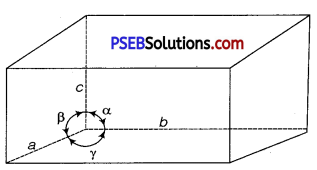(ii) angles between the edges, which are α (between b and c), β (between a and c) and γ(between a and b)

Question 12.
Distinguish between
(i) Hexagonal and monoclinic unit cells
(ii) Face-centred and end-centred unit cells.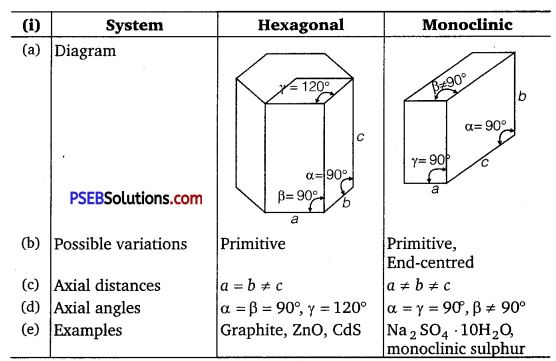Unit cell Face-centred End-centred (a) Position of lattice points At the corners and at the centre of each face At the corners and at the centres of two end faces (b) No. of atoms per unit cell 8 × $$\frac{1}{8}$$ + 6 × $$\frac{1}{2}$$ = 4 8 × $$\frac{1}{8}$$ + 2 × $$\frac{1}{2}$$ = 2

Question 13.
Explain how much portion of an atom located at (i)comer and
(ii) body-centre of a cubic unit cell is part of its neighbouring unit cell.
(i) An atom located at the corner of a cubic unit cell is shared by eight unit cells.
Therefore, $$\frac{1}{8}$$th portion of the atom is shared by one unit cell.

(ii) An atom located at the body centre of a cubic unit cell is not shared by its neighbouring unit cell. Therefore, the atom belongs only to the unit cell in which it is present i.e., its contribution to the unit cell is one.

Question 14.
What is the two dimensional coordination number of a molecule in square close packed layer?In square close-packed layer, a molecule is in contact with four of its neighbours. Therefore, the two-dimensional coordination number of a molecule in square close-packed layer is four.

Question 15.
A compound forms hexagonal close-packed structure. What is the total number of voids in 0.5 mol of it? How many of these are tetrahedral voids?
Solution:
Number of close-packed structure = 0.5 × 6.022 × 1023 = 3.011 × 1023
Therefore, number of octahedral voids = 3.011 × 1023
Number of tetrahedral voids = 2 × 3.011 × 1023 = 6.022 × 1023
Therefore, total number of voids
= 3.011 × 1023 + 6.022 × 1023 = 9.033 × 1023Question 16.
A compound is formed by two elements M and N. The element N forms ccp and atoms of M occupy 1/3rd of tetrahedral voids. What is the formula of the compound?
Solution:
Suppose atoms of element N represent in ccp = x
Then, number of tetrahedral voids = 2x
According to the question, the atoms of element M occupy $$\frac{1}{3}$$rd of the tetrahedral voids.
Therefore, the number of atoms of element M = 2x × $$\frac{1}{3}$$ = $$\frac{2 x}{3}$$
Ratio of M : N = $$\frac{2 x}{3}$$: x = 2 : 3
Thus, the formula of the compound is M2N3.

Question 17.
Which of the following lattices has the highest packing efficiency
(i) simple cubic
(ii) body-centred cubic and
(iii) hexagonal close-packed lattice?
Hexagonal close-packed lattice has the highest packing efficiency of 74%. The packing efficiencies of simple cubic and body-centred cubic lattices are 52.4% and 68% respectively.

Question 18.
An element with molar mass 2.7 × 10-2 kg mol-1 forms a cubic unit cell with edge length 405 pm. If its density is 2.7 × 103 kgm-3, what is the nature of the cubic unit cell?
Solution:
Given density, d = 2.7 × 103 kg m-3
Molar mass, M =2.7 × 10-2 kg mol-1
Edge length, a = 405 pm = 405 × 10-12m = 4.05 × 10-10 m
Avogadro’s number, NA = 6.022 × 1023 mol-1
Using the formula d = $$\frac{z \times M}{a^{3} \times N_{A}}$$ => z = $$\frac{d \times a^{3} \times N_{A}}{M}$$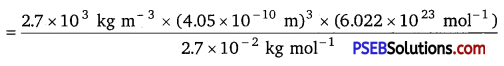= 4.004 = 4
Since, there are four atoms of the element present per unit cell. Hence, the cubic unit cell is face-centred cubic {fee) or cubic close-packed (ccp).

Question 19.
What type of defect can arise when a solid is heated? Which physical property is affected by it and in what way?
When a solid is heated, vacancy defect can arise. A solid crystal is said to have vacancy defect when some of the lattice sites are vacant. Vacancy defect leads to a decrease in the density of the solid.

Question 20.
What type of stoichiometric defect is shown’by:
(i) ZnS
(ii) AgBr
(i) ZnS shows Frenkel defect because its ions have large difference in size.
(ii) AgBr shows Frenkel defect as well as Schottky defect.Question 21.
Explain how vacancies are introduced in an ionic solid when a cation of higher valence is added as an impurity in it.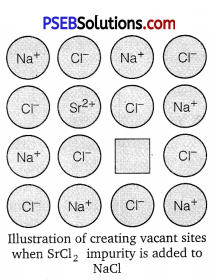When a cation of higher valence is added to an ionic solid as an impurity to it, the cation of higher valence replaces more than one cation of lower valence so as to keep the crystal electrically neutral. As a result, some sites become vacant. For example, when Sr2+is added to NaCl, each Sr2+ ion replaces two Na+ ions. However, one Sr2+ ion occupies the site of one Na+ ion and the other site remains vacant. Hence, vacancies are introduced. The reason is that the crystal as a whole is to remain electrically neutral.

Question 22.
Ionic solids, which have anionic vacancies due to metal excess defect, develop colour. Explain with the help of a suitable example.
The colour develops because of the presence of electrons in the anionic sites. These electrons absorb energy from the visible part of radiation and get excited. For example, when crystals of NaCl are heated in an atmosphere of sodium vapours, the sodium atoms get deposited on the surface of the crystal and the chloride ions from the crystal diffuse to the surface to form NaCl with the deposited Na atoms. During this process, the Na atoms on the surface lose electrons to form Na+ ions and the released electrons diffuse into the crystal to occupy the vacant anionic sites. These electrons get excited by absorbing energy from the visible light and impart yellow colour to the crystals. These electrons are called F-centres (from the German word Farbenzenter meaning colour centre).

Question 23.
A group 14 element is to be converted into re-type semiconductor by doping it with a suitable impurity. To which group should this impurity belong?